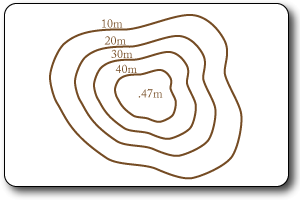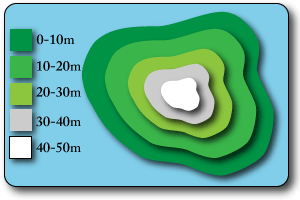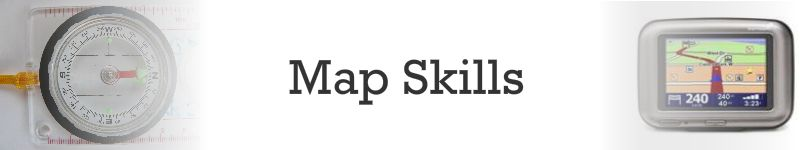### 4-Figure Grid References

These provide a reference telling us which grid square the object is in.

Go along the bottom first & up the side second:

• (01,10) = orange circle
• (02,11) = blue square
• (03,10) = green triangle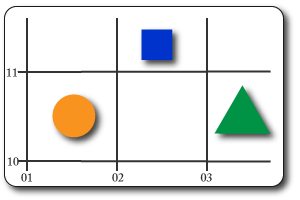### 6-Figure Grid References

These provide more accurate location by specifying the exact location within a grid square.

As before, go along the bottom to get the square & then put how many tenths across it is (like a decimal point). Normally you will have to do this bit in your head as the only the numbered lines will be shown.

Repeat this on the verticle axis.

• (014,105) = orange circle
• (023,111) = blue square
• (038,101) = green triangle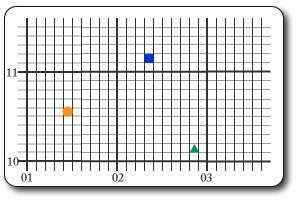### Contour Lines

These are the brown lines & they run along the sections of the map that are the same height. They are given a number which represents the height above sea level in meters. The closer together they are the steeper the gradient.

### Spot Heights

These are the exact height of a point shown with a black dot & the height in meters next to it.